# Fourier-Borel transform

(diff) ← Older revision | Latest revision (diff) | Newer revision → (diff)

Letbe the-dimensional complex space, and let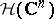denote the space of entire functions incomplex variables, equipped with the topology of uniform convergence on the compact subsets of(cf. also Entire function; Uniform convergence). Letbe its dual space of continuous linear functionals. The elements ofare usually called analytic functionals in.

One says that a compact set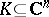is a carrier for an analytic functionalif for every open neighbourhoodofthere exists a positive constant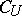such that, for every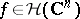,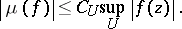General references for these notions are [a3], [a5].

Let. The Fourier–Borel transformis defined bywhere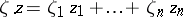For, the use of this transform goes back to E. Borel, while forit first appeared in a series of papers by A. Martineau, culminating with [a6].

It is immediate to show thatis an entire function. Moreover, since the exponentials are dense in, an analytic functional is uniquely determined by its Fourier–Borel transform.

By using the definition of carrier of an analytic functional, it is easy to see that if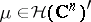is carried by a compact convex set, then for everythere exists a number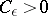such that, for any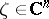,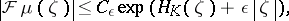where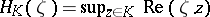is the support function of.

A fundamental result in the theory of the Fourier–Borel transform is the fact that the converse is true as well: Let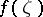be an entire function. Suppose that for some compact convex setand for everythere exists a numbersuch that, for any,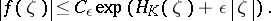(a1)

Thenis the Fourier–Borel transform of an analytic functionalcarried by.

This theorem, for, was proved by G. Pólya, while forit is due to A. Martineau [a7].

In particular, the Fourier–Borel transform establishes an isomorphism between the spaceand the space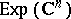of entire functions of exponential type, i.e. those entire functionsfor which there are positive constants,such that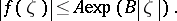If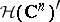is endowed with the strong topology, andwith its natural inductive limit topology, then the Fourier–Borel transform is actually a topological isomorphism, [a2].

A case of particular interest occurs when, in the above assertion, one takes. In this case, a function which satisfies the estimate (a1), i.e.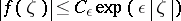is said to be of exponential type zero, or of infra-exponential type. Given such a function, there exists a unique analytic functionalsuch that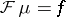; such a functional is carried byand therefore is a continuous linear functional on any space, foran open subset ofcontaining the origin. If one denotes by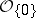the space of germs of holomorphic functions at the origin (cf. also Germ), then, the space of hyperfunctions supported at the origin (cf. also Hyperfunction); the Fourier–Borel transform is therefore well defined on such a space. In fact, it is well defined on every hyperfunction with compact support. For this and related topics, see e.g. [a1], [a4].

The Fourier–Borel transform is a central tool in the study of convolution equations in convex sets in. As an example, consider the problem of surjectivity. Letbe an open convex subset ofand letbe carried by a compact set. Then the convolution operator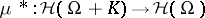is defined by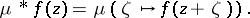One can show (see [a5] or [a1] and the references therein) that if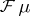is of completely regular growth and the radial regularized indicatrix ofcoincides with, thenis a surjective operator. The converse is true provided thatis bounded, strictly convex, withboundary.